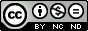## Publication Details

 Reference Category Journals DOI / URL link Creative Commons LicenceTitle (Primary) From modular decomposition trees to rooted median graphs Author Bruckmann, C.; Stadler, P.F.; Hellmuth, M. Journal Discrete Applied Mathematics Year 2022 Department UMB Volume 310 Page From 1 Page To 9 Language englisch Topic T7 Bioeconomy Keywords 2-structures; Symbolic ultrametrics; Modular decomposition; Prime module; Prime vertex replacement; Median graph; Algorithm; Half-grid; Hypercube Abstract The modular decomposition of a symmetric map delta:X x X -> Upsilon (or, equivalently, a set of pairwise-disjoint symmetric binary relations, a 2-structure, or an edge-colored undirected graph) is a natural construction to capture key features of delta in terms of a labeled tree. A map delta is explained by a vertex-labeled rooted tree (T, t) if the label delta(x, y) coincides with the label of the lowest common ancestor of x and y in T, i.e., if delta(x, y) = t(lca(x, y)). Only maps whose modular decomposition does not contain prime nodes, i.e., the symbolic ultrametrics, can be explained in this manner. Here we consider rooted median graphs as a generalization of (modular decomposition) trees to explain symmetric maps. We derive a linear-time algorithm that stepwisely resolves prime vertices in the modular decomposition tree to obtain a rooted and labeled median graph that explains a given symmetric map delta. Persistent UFZ Identifier https://www.ufz.de/index.php?en=20939&ufzPublicationIdentifier=26884 Bruckmann, C., Stadler, P.F., Hellmuth, M. (2022): From modular decomposition trees to rooted median graphs Discrete Appl. Math. 310 , 1 - 9